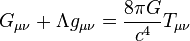# Quantum gravity facts for kids

Kids Encyclopedia Facts
General relativity$G_{\mu \nu} + \Lambda g_{\mu \nu}= {8\pi G\over c^4} T_{\mu \nu}$
Einstein field equations

Quantum gravity is an area of research in physics. Right now, physics is able to describe gravity of real-life sized objects using general relativity. Physicists can also describe the universe on a microscopic scale with quantum mechanics. The goal of quantum gravity is to develop a theory which contains both general relativity and quantum mechanics. However, combining the theories of the big and the small is very difficult in practice.

Physicists are working on a number of ways to write down a theory of quantum gravity. One approach is to search for a unified field theory. Physicists also try to develop theories at an even more fundamental level, like string theory, loop quantum gravity, or other methods, to find out why our current theories of gravity and quantum mechanics cannot be combined.

## Images for kidsQuantum gravity Facts for Kids. Kiddle Encyclopedia.